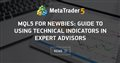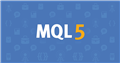# MQL5 Created multiple instance of QQE indicator on chart during backtest48

Hello and hope all are doing well.

I have tried to use QQE as external indicator into my EA

I have tried multiple way to load QQE with default value and also pass the correct values, in both cases during test it shows MULTIPLE instances of QQE on chart.

1- First way
```#define name_of_indicators "QQE"
#resource "\\Indicators\\QQE.ex5"

int OnInit()
{
//---
return(INIT_SUCCEEDED);
}
//+------------------------------------------------------------------+
//| Expert deinitialization function                                 |
//+------------------------------------------------------------------+
void OnDeinit(const int reason)
{
}
//+------------------------------------------------------------------+
//| Expert tick function                                             |
//+------------------------------------------------------------------+

void OnTick()
{
datetime DateTime = TimeCurrent();

MqlDateTime StrucTimeCurrent;
TimeToStruct(DateTime, StrucTimeCurrent);
if(StrucTimeCurrent.hour ==23 && StrucTimeCurrent.sec == 10)
{
Print("QQEFAST VALUE IS", GetQQE());
}
}

// ----------------------------------------
double GetQQE()
{
int QQE = getQQEHandle();

double QQEFast[];
ArraySetAsSeries(QQEFast, true);
CopyBuffer(QQE,1,0,5,QQEFast);
double Qqe= QQEFast;
return Qqe;
}

// ---------------------------------------
int getQQEHandle()
{
int QQE = iCustom(_Symbol,PERIOD_H1,name_of_indicators);

return QQE;
}```

2- Second way

```//+------------------------------------------------------------------+
//|                                                      ProjectName |
//|                                       http://www.companyname.net |
//+------------------------------------------------------------------+

#define name_of_indicators "QQE"
#resource "\\Indicators\\QQE.ex5"
#include <Indicators\Custom.mqh>

CiCustom qqe_indicator;
//+------------------------------------------------------------------+
//|                                                                  |
//+------------------------------------------------------------------+
int OnInit()
{
//---
return(INIT_SUCCEEDED);
}
//+------------------------------------------------------------------+
//| Expert deinitialization function                                 |
//+------------------------------------------------------------------+
void OnDeinit(const int reason)
{
}
//+------------------------------------------------------------------+
//| Expert tick function                                             |
//+------------------------------------------------------------------+

//+------------------------------------------------------------------+
//|                                                                  |
//+------------------------------------------------------------------+
void OnTick()
{
datetime DateTime = TimeCurrent();

MqlDateTime StrucTimeCurrent;
TimeToStruct(DateTime, StrucTimeCurrent);
if(StrucTimeCurrent.hour ==23 && StrucTimeCurrent.sec == 10)
{
Print("QQEFAST VALUE IS", GetQQE());
}
}

// ----------------------------------------
double GetQQE()
{
int qqe_handle;
MqlParam qqe_param[];
ArrayResize(qqe_param,8);

qqe_param.type         =TYPE_STRING;
qqe_param.string_value=name_of_indicators;

qqe_param.type         =TYPE_INT;
qqe_param.integer_value=14;

qqe_param.type         =TYPE_INT;
qqe_param.integer_value=5;

qqe_param.type         =TYPE_DOUBLE;
qqe_param.double_value=2.618;

qqe_param.type         =TYPE_DOUBLE;
qqe_param.double_value=4.236;

qqe_param.type         =TYPE_DOUBLE;
qqe_param.double_value=60;

qqe_param.type         =TYPE_DOUBLE;
qqe_param.double_value=40;

qqe_param.type         =TYPE_INT;
qqe_param.integer_value=PRICE_CLOSE;

qqe_handle = qqe_indicator.Create(_Symbol,PERIOD_H1,IND_CUSTOM,8,qqe_param);

if(!qqe_handle)
{
printf(__FUNCTION__+": error initializing object");
return(false);
}

double QQEbuffer[];
double qqe1 = qqe_indicator.GetData(0,5,3,QQEbuffer);

return qqe1;
}
//+------------------------------------------------------------------+
```

I have read this https://www.mql5.com/en/articles/31 and this https://www.mql5.com/en/forum/217721 page but still could find the why its show multiple instances.

**************  QQE indicator ***********

```//+------------------------------------------------------------------
#property description "QQE"

//+------------------------------------------------------------------
#property indicator_separate_window
#property indicator_buffers 7
#property indicator_plots   5
#property indicator_label1  "QQE histogram"
#property indicator_type1   DRAW_COLOR_HISTOGRAM2
#property indicator_color1  clrGainsboro,clrDeepSkyBlue,clrLightSalmon
#property indicator_width1  2
#property indicator_label2  "QQE fast"
#property indicator_type2   DRAW_LINE
#property indicator_color2  clrDarkGray
#property indicator_style2  STYLE_DOT
#property indicator_label3  "QQE slow"
#property indicator_type3   DRAW_LINE
#property indicator_color3  clrDarkGray
#property indicator_label4  "QQE"
#property indicator_type4   DRAW_COLOR_LINE
#property indicator_color4  clrDarkGray,clrDeepSkyBlue,clrLightSalmon
#property indicator_width4  2
//--- input parameters
input int                inpRsiPeriod          = 14;          // RSI period
input int                inpRsiSmoothingFactor =  5;          // RSI smoothing factor
input double             inpWPFast             = 2.618;       // Fast period
input double             inpWPSlow             = 4.236;       // Slow period
input double             inpUpBound            =  60;         // Upper bound
input double             inpDnBound            =  40;         // Lower bound
input ENUM_APPLIED_PRICE inpPrice              = PRICE_CLOSE; // Price
//--- buffers declarations
double val[],valc[],levs[],levf[],histoh[],histol[],histoc[];
//+------------------------------------------------------------------+
//| Custom indicator initialization function                         |
//+------------------------------------------------------------------+
int OnInit()
{
//--- indicator buffers mapping
SetIndexBuffer(0,histoh,INDICATOR_DATA);
SetIndexBuffer(1,histol,INDICATOR_DATA);
SetIndexBuffer(2,histoc,INDICATOR_COLOR_INDEX);
SetIndexBuffer(3,levf,INDICATOR_DATA);
SetIndexBuffer(4,levs,INDICATOR_DATA);
SetIndexBuffer(5,val,INDICATOR_DATA);
SetIndexBuffer(6,valc,INDICATOR_COLOR_INDEX);
IndicatorSetInteger(INDICATOR_LEVELS,2);
IndicatorSetDouble(INDICATOR_LEVELVALUE,0,inpUpBound);
IndicatorSetDouble(INDICATOR_LEVELVALUE,1,inpDnBound);
//--- indicator short name assignment
IndicatorSetString(INDICATOR_SHORTNAME,"QQE histo ("+(string)inpRsiPeriod+","+(string)inpRsiSmoothingFactor+")");
//---
return (INIT_SUCCEEDED);
}
//+------------------------------------------------------------------+
//| Custom indicator de-initialization function                      |
//+------------------------------------------------------------------+
void OnDeinit(const int reason)
{
}
//+------------------------------------------------------------------+
//| Custom indicator iteration function                              |
//+------------------------------------------------------------------+
int OnCalculate(const int rates_total,const int prev_calculated,const datetime &time[],
const double &open[],
const double &high[],
const double &low[],
const double &close[],
const long &tick_volume[],
const long &volume[],
{
if(Bars(_Symbol,_Period)<rates_total) return(prev_calculated);
int i=(int)MathMax(prev_calculated-1,0); for(; i<rates_total && !_StopFlag; i++)
{
val[i]=iEma(iRsi(getPrice(inpPrice,open,close,high,low,i,rates_total),inpRsiPeriod,i,rates_total),inpRsiSmoothingFactor,i,rates_total,0);
double _iEma = iEma((i>0 ? MathAbs(val[i-1]-val[i]) : 0),inpRsiPeriod,i,rates_total,1);
double _iEmm = iEma(                               _iEma,inpRsiPeriod,i,rates_total,2);
double _iEmf = _iEmm*inpWPFast;
double _iEms = _iEmm*inpWPSlow;
//
//---
//
{
double tr = (i>0) ? levs[i-1] : 0;
double dv = tr;
if(val[i] < tr) { tr = val[i] + _iEms; if((i>0 && val[i-1] < dv) && (tr > dv)) tr = dv; }
if(val[i] > tr) { tr = val[i] - _iEms; if((i>0 && val[i-1] > dv) && (tr < dv)) tr = dv; }
levs[i]=tr;
}
{
double tr = (i>0) ? levf[i-1] : 0;
double dv = tr;
if(val[i] < tr) { tr = val[i] + _iEmf; if((i>0 && val[i-1] < dv) && (tr > dv)) tr = dv; }
if(val[i] > tr) { tr = val[i] - _iEmf; if((i>0 && val[i-1] > dv) && (tr < dv)) tr = dv; }
levf[i]=tr;
}
histoh[i] = val[i];
histol[i] = 50;
valc[i]   = (val[i]>levf[i] && val[i]>levs[i]) ? 1 :(val[i]<levf[i] && val[i]<levs[i]) ? 2 :(i>0) ? valc[i-1]: 0;
histoc[i] = (val[i]>inpUpBound) ? 1 :(val[i]<inpDnBound) ? 2 :0;
}
return (i);
}
//+------------------------------------------------------------------+
//| Custom functions                                                 |
//+------------------------------------------------------------------+
#define rsiInstances 1
#define rsiInstancesSize 3
double workRsi[][rsiInstances*rsiInstancesSize];
#define _price  0
#define _change 1
#define _changa 2
//
//---
//
double iRsi(double price,double period,int r,int bars,int instanceNo=0)
{
if(ArrayRange(workRsi,0)!=bars) ArrayResize(workRsi,bars);
int z=instanceNo*rsiInstancesSize;

//
//
//
//
//

workRsi[r][z+_price]=price;
if(r<period)
{
int k; double sum=0; for(k=0; k<period && (r-k-1)>=0; k++) sum+=MathAbs(workRsi[r-k][z+_price]-workRsi[r-k-1][z+_price]);
workRsi[r][z+_change] = (workRsi[r][z+_price]-workRsi[z+_price])/MathMax(k,1);
workRsi[r][z+_changa] =                                         sum/MathMax(k,1);
}
else
{
double alpha=1.0/MathMax(period,1);
double change=workRsi[r][z+_price]-workRsi[r-1][z+_price];
workRsi[r][z+_change] = workRsi[r-1][z+_change] + alpha*(        change  - workRsi[r-1][z+_change]);
workRsi[r][z+_changa] = workRsi[r-1][z+_changa] + alpha*(MathAbs(change) - workRsi[r-1][z+_changa]);
}
return(50.0*(workRsi[r][z+_change]/MathMax(workRsi[r][z+_changa],DBL_MIN)+1));
}
//
//---
//
double workEma[];
//
//---
//
double iEma(double price,double period,int r,int bars,int instanceNo=0)
{
if(ArrayRange(workEma,0)!=bars) ArrayResize(workEma,bars);

//
//---
//

workEma[r][instanceNo]=price;
if(r>0 && period>1)
workEma[r][instanceNo]=workEma[r-1][instanceNo]+2.0/(1.0+period)*(price-workEma[r-1][instanceNo]);
return(workEma[r][instanceNo]);
}
//
//---
//
double getPrice(ENUM_APPLIED_PRICE tprice,const double &open[],const double &close[],const double &high[],const double &low[],int i,int _bars)
{
switch(tprice)
{
case PRICE_CLOSE:     return(close[i]);
case PRICE_OPEN:      return(open[i]);
case PRICE_HIGH:      return(high[i]);
case PRICE_LOW:       return(low[i]);
case PRICE_MEDIAN:    return((high[i]+low[i])/2.0);
case PRICE_TYPICAL:   return((high[i]+low[i]+close[i])/3.0);
case PRICE_WEIGHTED:  return((high[i]+low[i]+close[i]+close[i])/4.0);
}
return(0);
}
//+------------------------------------------------------------------+
```MQL5 for Newbies: Guide to Using Technical Indicators in Expert Advisors
• www.mql5.com
An Expert Advisor or indicator that doesn't use standard technical indicators in its code is rare. They are popular both for the beginners and advanced developers of trading strategies. It isn't difficult to understand the details of indicator creation; the aim of this article is to help with it. We will consider the use of the functions for...39066

You are executing iCustom on each tick, each time it creates an instance of the indicator. However if you read the article you mentioned yourself, it emphasis clearly :

The handle of the indicator should be created inside the function OnInit()

So what's the point to "read" an article ?48

Alain Verleyen:

You are executing iCustom on each tick, each time it creates an instance of the indicator. However if you read the article you mentioned yourself, it emphasis clearly :

So what's the point to "read" an article ?

First of all, Thanks for you response.

Yes you are right and I did that before and no issue about that I would like to have value of QQE in different Timeframe and different Symbol during my test. Should I create different handle for different Timeframe and Symbol OnInit() function and use it?

Imagine I want to have value of QQE for These Timeframe H4, H1, M30, M15 since I have to pass the Timeframe as input for iCustom function as param. it means I should have 4 different handle for QQE and what if I want to see that value in other Timeframes and Symbol?

I don't think so its correct please let me know how you tackle this issue dear Alain.

```int QQE_H4 = iCustom(_Symbol,PERIOD_H4,name_of_indicators);
int QQE_H1 = iCustom(_Symbol,PERIOD_H1,name_of_indicators);
int QQE_M30 = iCustom(_Symbol,PERIOD_M30,name_of_indicators);
int QQE_M15 = iCustom(_Symbol,PERIOD_M15,name_of_indicators);

```Documentation on MQL5: Constants, Enumerations and Structures / Named Constants / Predefined Macro Substitutions
• www.mql5.com
//| Expert initialization function                                   | //| Expert deinitialization function                                 | //| Expert tick function                                             | //| test1                                                            |...39066

HF2020:

First of all, Thanks for you response.

Yes you are right and I did that before and no issue about that I would like to have value of QQE in different Timeframe and different Symbol during my test. Should I create different handle for different Timeframe and Symbol OnInit() function and use it?

Imagine I want to have value of QQE for These Timeframe H4, H1, M30, M15 since I have to pass the Timeframe as input for iCustom function as param. it means I should have 4 different handle for QQE and what if I want to see that value in other Timeframes and Symbol?

I don't think so its correct please let me know how you tackle this issue dear Alain.

Yes you need one handle for each timeframe and you need to do it in OnInit().48

Alain Verleyen:
Yes you need one handle for each timeframe and you need to do it in OnInit().

Thanks man :)